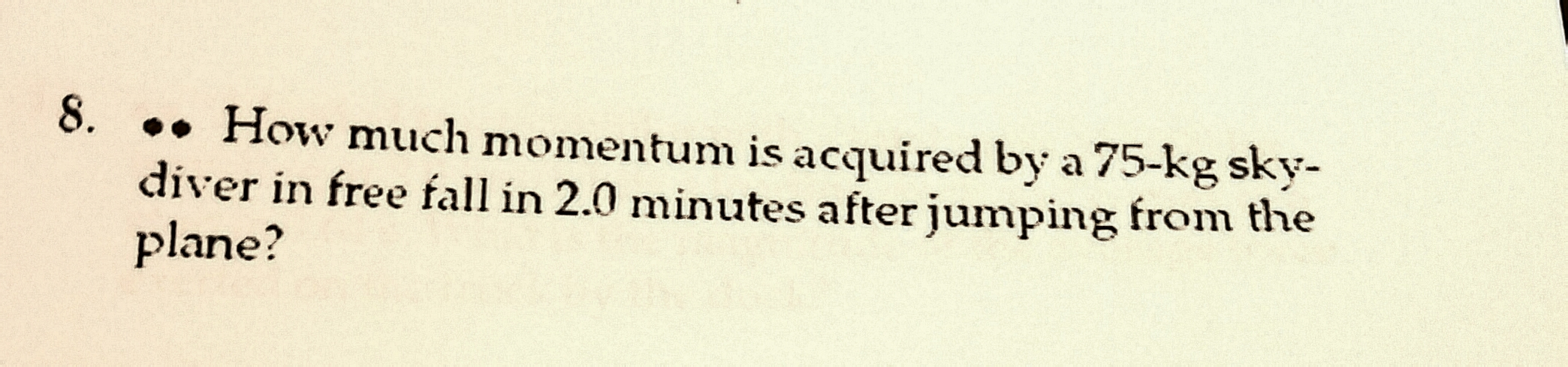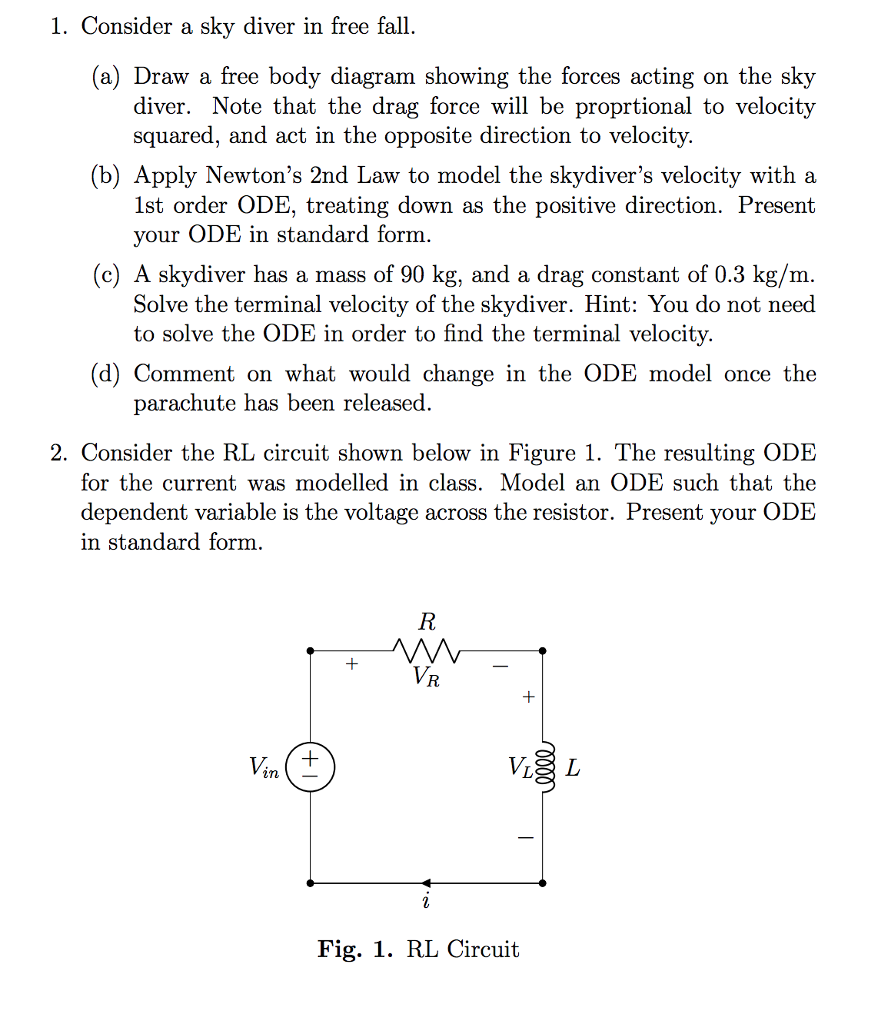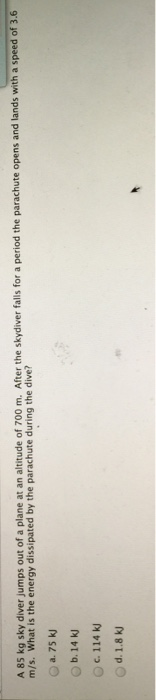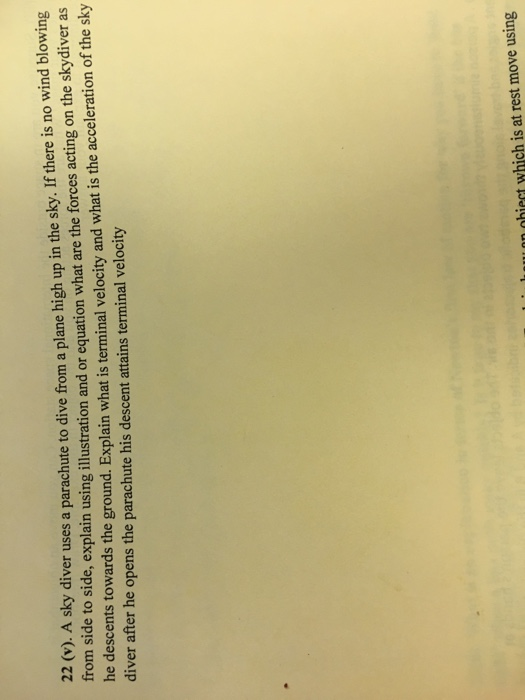Question

How much momentum is acquired by a 75-kg sky-diver in free fall in 2.0 minutes after...How much momentum is acquired by a 75-kg sky-diver in free fall in 2.0 minutes after jumping from the plane?

step:1

Given data

mass m=75 kg

time t=2 min

step:2

use the kinematices to find the velocity driver

=>v-u=gt

=>v=9.8*2*60=1176 m/s

the velocity of driver falls v=1176 m/s

step:3

now we find the momentum

momentum P=mv=75*1176=88200 kg.m/s

SOLUTION :

Velocity of the driver 2 minute after jumping, v :

= g * (2 *60)

= 9.8 * 120

= 1176 m/s

So, momentum acquired by the driver at the instant

= m * v

= 75 * 1176

= 88200 kg - m / s.  (ANSWER).

Earn Coins

Coins can be redeemed for fabulous gifts.

Similar Homework Help Questions
• 1. Consider a sky diver in free fall (a) Draw a free body diagram showing the...1. Consider a sky diver in free fall (a) Draw a free body diagram showing the forces acting on the sky diver. Note that the drag force will be proprtional to velocity squared, and act in the opposite direction to velocity. (b) Apply Newton's 2nd Law to model the skydiver's velocity with a 1st order ODE, treating down as the positive direction. Present your ODE in standard form (c) A skydiver has a mass of 90 kg, and a drag...

• sky diver

A sky diver of mass 80.0 (includingparachute) jumps off a plane and begins her descent.Throughout this problem use 9.80 for the magnitude of the acceleration due to gravity.Part A: At some point during her free fall, the sky diver reaches her terminal speed. What is the magnitude of the drag force due to air resistance that acts on the sky diver when she hasreached terminal speed?Express your answer in newtons.Part B: For an object falling through air at a high...

• A 85 kg sky diver jumps out of a plane at an altitude of 700 m....A 85 kg sky diver jumps out of a plane at an altitude of 700 m. After the skydiver falls for a period the parachute opens and lands with a speed of 3.6 m/s. What is the energy dissipated by the parachute during the dive? 75 kJ 14 kJ 114 kJ 1.8 kJ

• When sky divers initially fall from an airplane their velocity v in miles per hour after free falling d feet can be approximated by v=60/11√d

When sky divers initially fall from an airplane their velocity v in miles per hour after free falling d feet can be approximated by v=60/11√d. (Because of air resistance, they will eventually reach terminal velocity.) How far do sky divers need to fall to attain a velocity of 55 miles per hour? (This value for d represents the minimum distance.)A sky diver needs to fall about ? feet to attain a velocity of 55mph.

• Can you draw a free body diagram and explain this question...please&thankyou 22 (v). A sky diver...Can you draw a free body diagram and explain this question...please&thankyou 22 (v). A sky diver uses a parachute to dive from a plane high up in the sky. If there is no wind blowing from side to side, explain using illustration and or equation what are the forces acting on the skydiver as he descents towards the ground. Explain what is terminal velocity and what is the acceleration of the sky diver after he opens the parachute his descent...

• The function s(t) = 16t^2 models the distance, s(t), in feet, that an object falls in t seconds

The function s(t) = 16t^2 models the distance, s(t), in feet, that an object falls in t seconds. Find the number of seconds a sky diver is in free fall after jumping from a plane if she falls 192 feet before opening a parachute. Express your answer in simplified radical form.

Dear Cramster Friends,Below is a challenge that I encountered but to which theanswer is not given. Can you please see if I have attemptedcorrectly? I urge you to please not be put offby the lenght of thequestion. Whilst I don't deny that the question has too many parts,the truth of the matter is that it won't take long at all.Thank-you verymuch for your time and effort.(Q) A sky diver of mass 80.0 (including parachute) jumps off a plane and begins...

• neglecting wind restitance,the height,h,in feet,of a sky diver,t,seconds after she jumps from an airplane 1,648 above the ground is given by the formula h=-16ft square +1648

neglecting wind restitance,the height,h,in feet,of a sky diver,t,seconds after she jumps from an airplane 1,648 above the ground is given by the formula h=-16ft square +1648.if the parachute opens 4.5 secinds after the sky diver leaveshe airplane,about how far will she be from the ground when the parchute opens? will it be -16 x 4.5 square + 1648=1324?if not may you show me the correct answer and solve please.

• After a fall, a 91 kg rock climber finds himself dangling from the end of a...

After a fall, a 91 kg rock climber finds himself dangling from the end of a rope that had been 18 m long and 8.0 mm in diameter but has stretched by 2.0 cm. For the rope, calculate (a) the strain, (b) the stress, and (c) the Young's modulus.

• A friction-free, weighted piston-cylinder device contains 2.0 kg of water in a mixed state at a...

A friction-free, weighted piston-cylinder device contains 2.0 kg of water in a mixed state at a pressure of 150kPa and a quality of 0.60. As it sits there, heat is lost until the mixture becomes entirely a saturated liquid. How much heat is lost in the process? Thanks!